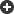# Line graph - Definition with Examples

The Complete Prek-5 Learning Program Built for Your Child
Home > Line graph

What is Line Graph?

A line graph is a type of chart used to show information that changes over time. We plot line graphs using several points connected by straight lines.  We also call it a line chart. The line graph comprises of two axes known as ‘x’ axis and ‘y’ axis.

• The horizontal axis is known as the x-axis.
• The vertical axis is known as the y-axis.

### Parts of a line graph

The given image describes the parts of a line graph.

Title: tells about the data for each line graph is plotted.

x-axis: tells about labels on x-axis, which is generally time.

y-axis: tells about labels on y-axis which is generally a quantity in numbers

Trend: We join the points to draw the graph. The intersection point of the label on the x-axis and y-axis tells about the trend. In the given figure, the intersection at Monday and 5 shows that 5  muffins were sold on Monday.

### Plotting a line graph

Plotting a line graph is easy. Here are the simple steps to consider while plotting a line graph.

• Draw the x-axis and y-axis on the graph paper. Make sure to write the title above the table so that it determines the purpose of the graph.

• For instance, if one of the factors is time, it goes on the horizontal axis,  referred to as the x-axis. The other factor would subsequently go on the vertical axis, which is known as the y-axis. Label both the axes as per their respective factors. For example, we can label the x-axis as time or day.

• Afterward, with the help of the already given data, point out the exact values on the graph. Once you join the points, you can make a clear inference about the trend.

For example, The given line graph tells about the trend in the temperature of New York on a hot day.

Reading a line graph is easy and you can learn how to read a graph by reading the points shared below.

• First, take a look at the two axes and try to understand what these axes denote.

• Then look at the graph and examine the values of the points situated in the lines of the graph.

• Follow the lines and figure out if there is any kind of rise or fall. Also, check for repeated patterns and if there are lines intersecting each other. This way, you will get to know the purpose of the line graph.

• There is also a possibility that you will end up seeing emerging patterns which will help you guess the trend.

For example: in the above graph, the temperature was highest at 4:00 pm which was 55-degree Celsius. Read the above graph and answer the following questions:

A: What is this graph about?

B: When was the temperature minimum?

C: What was the temperature at 3:00 pm?

 Fun Facts about Line Graph The initial line graphs were made by various persons namely Nicolaus Samuel Cruquius, Francis Hauksbee, William Playfair and Johann Heinrich Lambert.

•Can there be more than one line in a line graph?

Yes, there can be more than one line in a line graph. This is usually done when we need to compare two sets of information represented by each line.

•Where do we use a line graph in real-life?

Line graphs are used to track changes over short and long periods. Line graphs are useful to study or represent temperature, rainfall, the revenue of a company, etc.

•Do we always get a straight line in a line graph?

No. The line formed by joining all the data points in a line graph may or may not be straight. The line graph with a straight line is called a linear graph.

•What is a double line graph?

A double line graph is a type of line graph that represents two sets of data or information in a single graph. It is mainly used when we need to compare two sets of information and make inferences.

## Practice Problems on Line graphs